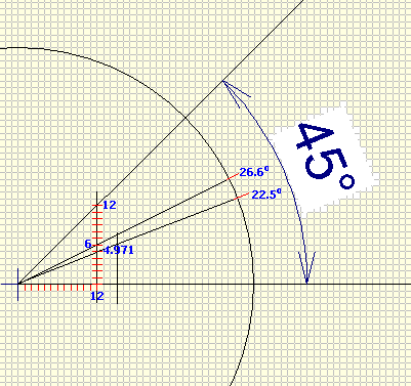Spike's Calculators

# Slope Ratio to Degrees

Convert a roof pitch of rise in 12 to a roof angle in degrees.

##### Example

With a 12 in 12 slope, the wall or roof rises 12 inches in a 12-inch run
A 12 in 12 slope = 45°

One could be inclined to assume that a 6 in 12 slope would equal half of that, 22.5°. A 6" rise in a 12-inch run. But that would be a wrong assumption!

You are not halving the angle by reducing the rise this way.

`A 6 in 12 slope = 26.5651 degrees, and 4.97056 in 12 slope = 22.5 degrees.`### Pitch to Angle

Slope :12

#### Results:

 Angle: °

#### The Calculation

1. enter the slope in inches per foot

#### Results

1. angle in degrees